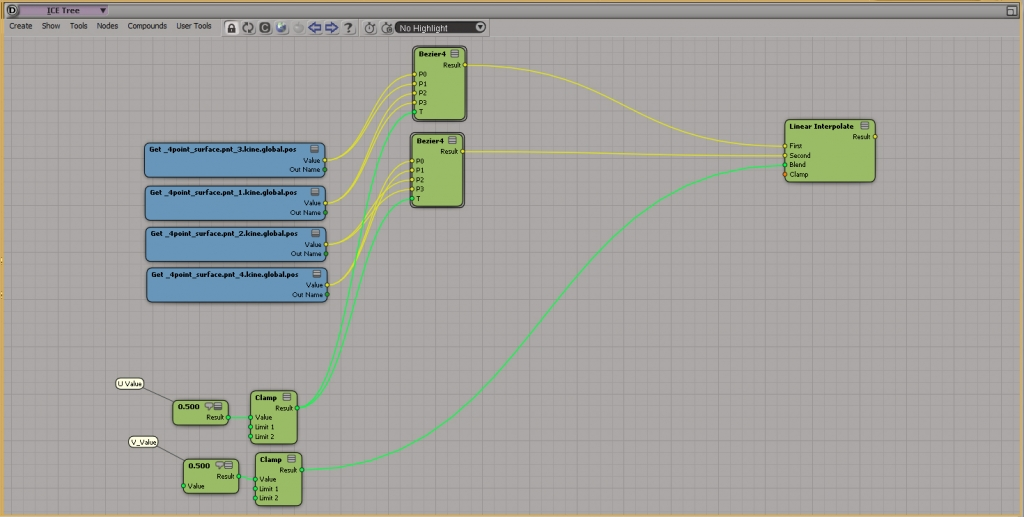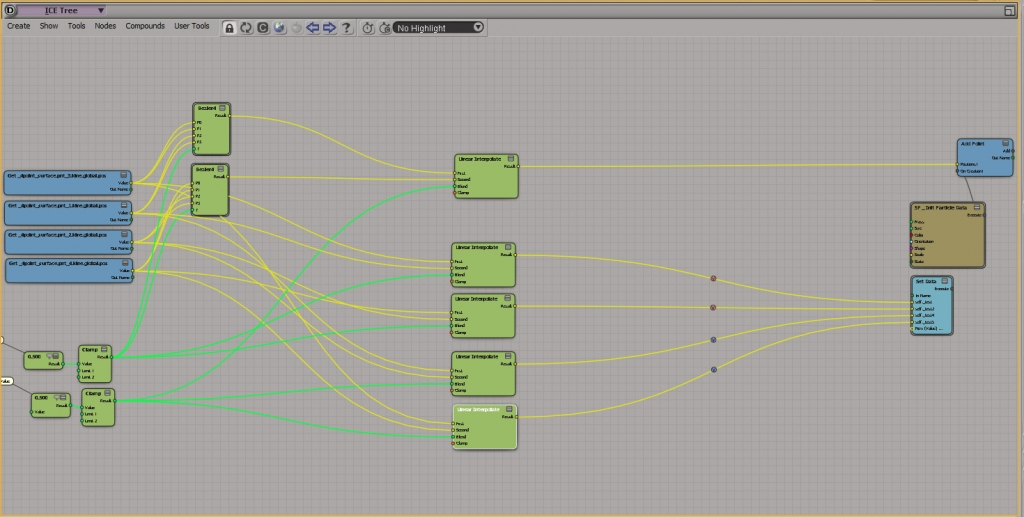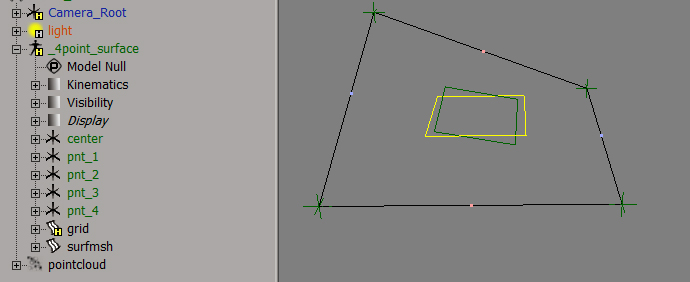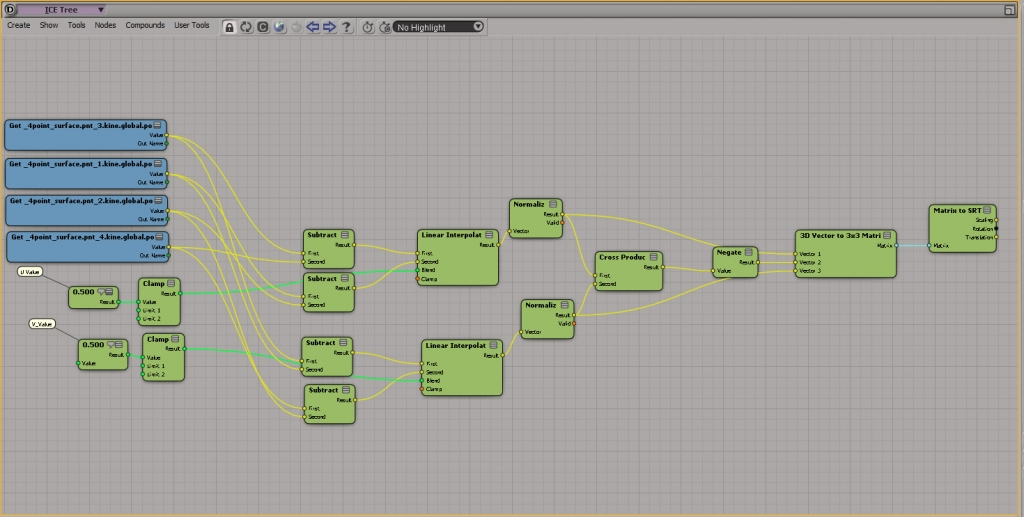# 4Point Surface Constraint in ICE

When rigging swimming or flowting things it’s not the best idea to put the hole object under one null and to rotate that. A better idea is to make a linear surface grid with four points, cluster constrain the four points to nulls and create a fifth null which is surface constrained to the middle of the surface.

As shown in the fist picture, the null moves/rotates nicely between the four control points. The principle is not new. There is also an Rigging solver of Studio Nest out there that does exact this.

Point Surface This solver computes a transform on a iso surface build by 2D bezier curves. These curves are defined by 4 controllers (the corners of the iso surface).

article: Sixbirds Rigging Solvers
backup: search for “Brigks”

When thinking of this case I asked myself how I would realize this within ICE without a real surface. I came up with a small graph that I try to explain.First of all we need the global positions of the four nulls.With that done we drop two “Bezier 4” nodes in. For thous of you who don’t know what the node is doing, read this Article on Wikipedia, and/or watch the video-tutorial of Bradley Gabe on Vimeo. That will clarify what this node is doing. Plugin in the Vectors as shown below. The order depents on position of the control nulls.Next we need two “Scalar” nodes for the U and V coordinate, two “Clamp” nodes and one “Linear Interpolate” node. Connect the output of the “Bezier 4” nodes to the inputs of the “Linear Interpolate” node. Then connect each “Scalar” node to one “Clamp” node and set the clamp-range from 0-1. With this way we make sure that the range is always right. Connect one of the “Clamp” nodes the the “Bezier 4” nodes (this will be our V coordinate weight), and the other one to the “Linear Interpolate” node weight (this will be our U coordinate weight). Ok, that’s all for the position logic.For visualization purposes I took a “Add Point” node, pluged in in the 3d position vector and initialized the basic particle attributes. Further more I used some linear interpolation nodes to visualize the current u and v on the grid. This ones are not necessary to get the Constraint working.The result of this graph looks like this. The ICE setup aligns perfectly the traditional surface constraint.  The blue points visualize the u coordinate and the red points the v coordinate. The only thing missing here is the proper rotation.The next step is a bit tricky and not THE solution, but I found this one  working best for me. The fist step is to get the vector for the U Coordinate by subtracting and interpolating. Second step is to do the same for the V  Coordinate. With that working we can calculate the third vector we are after with the “Cross Product” node.  For more information about that look at the Wikipedia article. By putting this three vectors in an  “3D Vector to 3×3 Matrix” node we can get an approximation of the rotation. Just resplit the Matrix back to SRT and plug the rotation in the  Particle Orientation Port.As shown below, the result lines up nicely to the traditional surface constraint.In some extreme cases, there are problems with the rotation. But for simple setups, this ICE graph could be an solution. Also it’s possible to drive Objects via ICE Kinematics with this method. I hope you like this small tutorial.